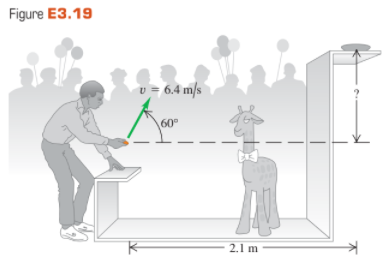×
Get Full Access to University Physics - 13 Edition - Chapter 3 - Problem 19e
Get Full Access to University Physics - 13 Edition - Chapter 3 - Problem 19e

×

# Win the Prize. In a carnival booth, you can win a stuffedISBN: 9780321675460 31

## Solution for problem 19E Chapter 3

University Physics | 13th Edition

• Textbook Solutions
• 2901 Step-by-step solutions solved by professors and subject experts
• Get 24/7 help from StudySoup virtual teaching assistantsUniversity Physics | 13th Edition

4 5 1 383 Reviews
11
0
Problem 19E

Win the Prize. In a carnival booth, you win a stuffed giraffe if you toss a quarter into a small dish. The dish is on a shelf above the point where the quarter leaves your hand and is a horizontal distance of $$2.1 \mathrm{~m}$$ from this point (Fig. E3.19). If you toss the coin with a velocity of $$6.4 \mathrm{~m} / \mathrm{s}$$ at an angle of $$60^{\circ}$$ above the horizontal, the coin lands in the dish. You can ignore air resistance. (a) What is the height of the shelf above the point where the quarter leaves your hand? (b) What is the vertical component of the velocity of the quarter just before it lands in the dish?Step-by-Step Solution:

Step 1 of 2

Velocity of projection $$=6.4 \mathrm{~m} / \mathrm{s}$$

The $$\mathrm{x}$$-component of velocity $$=6.4 \times \cos 60^{\circ}$$

$$v_{x}=3.2 \mathrm{~m} / \mathrm{s}$$

The $$y$$-component of velocity $$=6.4 \times \sin 60^{\circ}$$

$$v_{y}=5.54 \mathrm{~m} / \mathrm{s}$$

Horizontal distance $$=2.1 \mathrm{~m}$$

Let, $$t$$ be the time to cover this distance.

$$v_{x} t=2.1 \mathrm{~m}$$

$$t=2.1 / 3.2 \mathrm{~m} / \mathrm{s}$$

$$t=0.65 \mathrm{~s}$$

Step 2 of 2

##### ISBN: 9780321675460

The answer to “?Win the Prize. In a carnival booth, you win a stuffed giraffe if you toss a quarter into a small dish. The dish is on a shelf above the point where the quarter leaves your hand and is a horizontal distance of $$2.1 \mathrm{~m}$$ from this point (Fig. E3.19). If you toss the coin with a velocity of $$6.4 \mathrm{~m} / \mathrm{s}$$ at an angle of $$60^{\circ}$$ above the horizontal, the coin lands in the dish. You can ignore air resistance. (a) What is the height of the shelf above the point where the quarter leaves your hand? (b) What is the vertical component of the velocity of the quarter just before it lands in the dish?” is broken down into a number of easy to follow steps, and 117 words. The full step-by-step solution to problem: 19E from chapter: 3 was answered by , our top Physics solution expert on 05/06/17, 06:07PM. Since the solution to 19E from 3 chapter was answered, more than 3335 students have viewed the full step-by-step answer. This full solution covers the following key subjects: Quarter, dish, point, Win, shelf. This expansive textbook survival guide covers 26 chapters, and 2929 solutions. University Physics was written by and is associated to the ISBN: 9780321675460. This textbook survival guide was created for the textbook: University Physics, edition: 13.

## Discover and learn what students are asking

Calculus: Early Transcendental Functions : Exponential and Logarithmic Functions
?In Exercises 7-22, solve for $$x$$. $$4^{x}=64$$

Statistics: Informed Decisions Using Data : Inference about the Difference between Two Medians: Dependent Samples
?You Explain It! Dietary Habits A student in an exercise science program wishes to study dietary habits of married couples. She surveys married couples

Unlock Textbook Solution